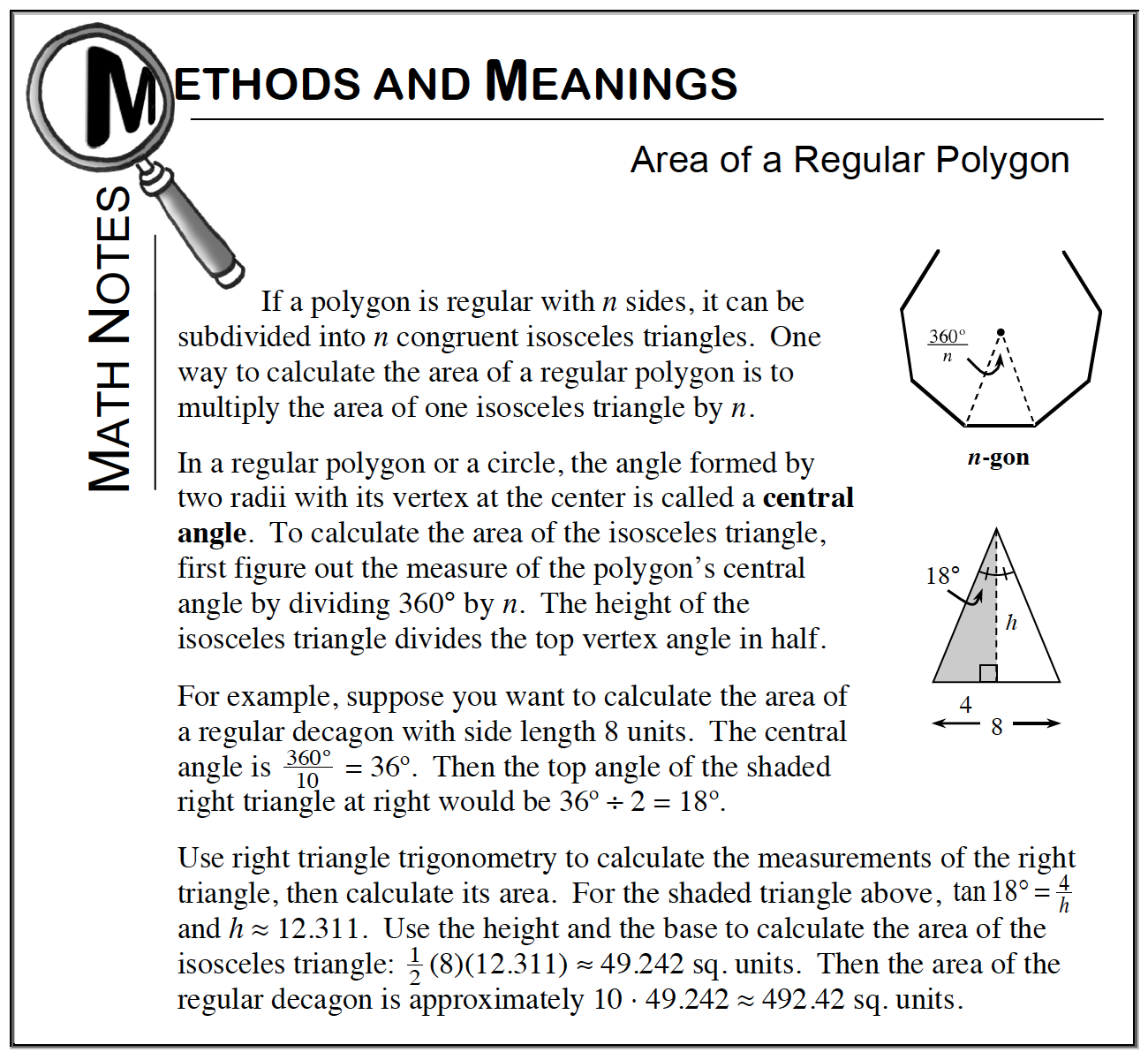### Home > CCG > Chapter 8 > Lesson 8.3.2 > Problem8-107

8-107.

An exterior angle of a regular polygon measures $18°$. Homework Help ✎

1. How many sides does the polygon have?

Remember that the sum of all exterior angles is equal to $360°$.

2. If the length of a side of the polygon is $2$ units, what is the area of the polygon?

Refer to the Math Notes box below, which gives a step-by-step example illustrating how to solve this type of problem.$≈ 126.3$ units$^2$